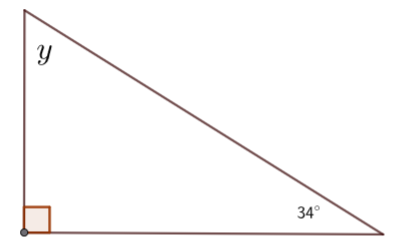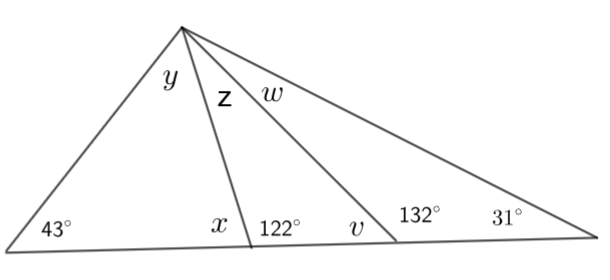Find the unknown angles in the figures below....... Answers to the Above Questions x = 61� y = 56� x = 36� , y = 124� , z = 20� y = 52� , x = 64� x = 14� , z = 47� , y = 19� w = 17� , v = 48� , z = 10� , x = 58� , y = 79�